### Lookup with multiple criteria's

This article was originally written for the Microsoft Excel Blog. The purpose of this article is to show Excel users some technique’s, and Excel functions, that they can use to lookup up a value on a table, using more than one criteria. If you have only one criteria, it’s simple, you can use a plain VLOOKUP formula to do that. If you want to use more than one, then there are lots of ways of doing it, using several of Excel’s functions like VLOOKUP, LOOKUP, MATCH, INDEX, etc.

Let’s see a scenario where we want to use two criteria’s to return a value from a table. This is the data table that we have: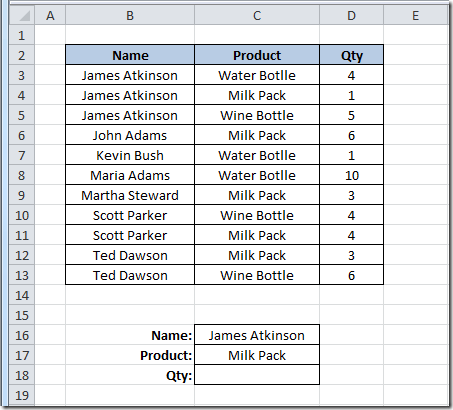As you can see, we want to use a “Name” and “Product” criteria to return a “Qty” value on cell C18. We are going to look for the Name “James Atkinson” and the Product “Milk Pack” to return the Qty from the table. Because the value that we want to return is a number, we can use a simple SUMPRODUCT() formula in C18 cell, like this:

=SUMPRODUCT((B3:B13=C16)*(C3:C13=C17)*(D3:D13))

What this does is look on the range B3:B13 for the value on cell C16 and on the range C3:C13 for the value on cell C17 and where it finds both, return the value on column D, from the same row where it found both criteria’s. Here’s how it will look: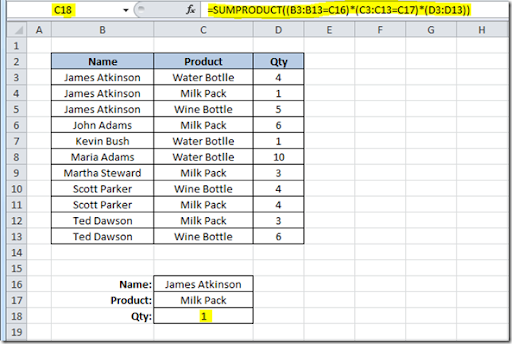It is returning the value 1 that corresponds to the value on cell D4 because it found “James Atkinson” Name on row 4 and also “Milk Pack” on the same row, thus returning the value of column D from that row. Let’s just change value on cell C5 from “Wine Bottle” to “Milk Pack” to see what happens with the formula on cell C18: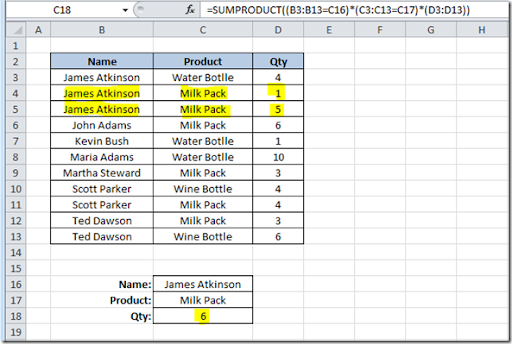Because our formula found two lines where both criteria’s were found, it is summing the values on column D on rows where they were found, giving us a Qty of 6.

This technique cannot be used is we wanted to look for two criteria’s and return a text result. For instance, this, would not work: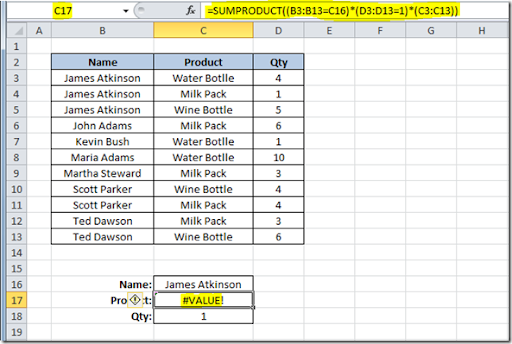We are looking for the Name “James Atkinson”, where the Qty is 1 and we want to return the Product name that matches this two criteria’s. This formula would give us a #VALUE error! Instead we could use a formula using a combination of SUMPRODUCT, INDEX and ROW functions, like this:

=INDEX(C3:C13,SUMPRODUCT((B3:B13=C16)*(D3:D13=C18)*ROW(C3:C13)),0)

We use the SUMPRODUCT function to find out the row where both criteria’s are found and return the corresponding row number using the ROW function. Then we use it on the INDEX function to return the value on the array C3:C13 that is on the row number provided. The result will be like this: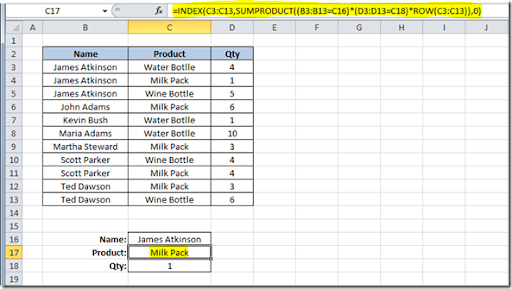This could also be done using a different technique. You could use this formula on cell C17:

=LOOKUP(2,1/(B3:B13=C16)/(D3:D13=C18),(C3:C13))

The result will be the same as the previous solution. What this formula does, is divide 1 by an array of True/False values (B3:B13=C16) and then by another array of True/False (D3:D13=C18). This will return 1 or a #DIV/0 error. Using 2 as the lookup value, then the match will be with the last numeric value in the range, i.e. the last row where both conditions are True. This will be our "vector form" of the LOOKUP, so then you get the corresponding value returned from C3:C13. I used 2 as the LOOKUP value but it can be any number, starting at 1. If the formulas don’t find any match, of course you will get a #N/A error!

You could also use an array formula, using the MATCH function, like this:

{=INDEX(C3:C13,MATCH(1,(B3:B13=C16)*(D3:D13=C18),0))}

On this technique, I used the MATCH function to find the row where both conditions are found. This will return a value of 1 that will be matched to the 1 that was used as the lookup value of the MATCH function, thus returning us the row where the conditions are met. Using the INDEX value, we look for the value that is on the range C3:C13, that is on the row that was returned from the MATCH function, in this case, it was row 2, that corresponds to the second row on C3:C13.

All of this examples show you how to use two criteria’s for your lookups. It’s easy to do it if you have more than two, just add then to your formulas. Here how the formulas presented before, would look if you add one more criteria:

=SUMPRODUCT((B3:B13=C16)*(C3:C13=C17)*(E3:E13=C18)*(D3:D13))

=INDEX(C3:C13,SUMPRODUCT((B3:B13=C16)*(D3:D13=C18)*(E3:E13=C18)*ROW(C3:C13)),0)

=LOOKUP(2,1/(B3:B13=C16)/(D3:D13=C18)/(E3:E13=C18),(C3:C13))

{=INDEX(C3:C13,MATCH(1,(B3:B13=C16)*(D3:D13=C18)*(E3:E13=C18),0))}

As you can see, depending on your data tables, you can use several different techniques to lookup your values, using different Excel functions. Hope that you can apply this to your own Excel sheets.

#### 22 comentários:

Ankit Machama said...

Hey this was a nice idea, I wasn't even aware that lookups can be based on multiple criteria.
It was a bit complicated but you just explained it nicely.
Thanks :)

Excel Maniac said...

Very useful article.
Delivers solution in easy to understand way. I can recommend it.

Warren said...

Thanks for this. Found this very useful today. The examples of how to extend the formula further are much appreciated.

by Gabriel Braun said...

=LOOKUP(2,1/(B3:B13=C16)/(D3:D13=C18)/(E3:E13=C18),(C3:C13))

I used 2 as the LOOKUP value but it can be any number, starting at 1.

Could you please explain LOOKUP value in more detail. It seems that found value has to be less than lookup value. ex if i used 2 for lookup value result would be N/A if the matching value 2.1 or greater

Loconinja said...

This example is incorrect and only appears to work:

=INDEX(C3:C13,SUMPRODUCT((B3:B13=C16)*(D3:D13=C18)*ROW(C3:C13)),0)

The intention is to return the value in C4 ("Milk Pack"), but it actually returns C6. As this is also "Milk Pack", it appears successful.

The ROW(C3:C13) array must begin at row 1 for the formula to return the intended result, for example: ROW(C1:C11).

Unknown said...

Good & explained very nicely. Thanks

Best Excel Tutorial said...

I've learnt something new here. Thanks!

obat kuat cialis 50mg said...
Anonymous said...

قنوات اخبارية عربية بث مباشر , شاهد قنوات اخبارية مباشرة على الانترنت اون لاين , قنوات اخبار عربية بث مباشر جودة عالية , شاهد القنوات الاخبارية العربية , قنوات عالمية بث مباشر , قنوات مصرية , قنوات عراقية , قنوات اخبارية على النايل سات و العرب سات , اسماء القنوات الاخبارية العربية والعالمية , شاهد البث المباشر للقنوات الاخبارية العربية من خلال موقعنا بجودة عالية شاهد قناة العالم قناة روسيا اليوم بي بي سي عربي يورو نيوز الحوار المستقلة فرانس 24 قناة الحرة آي24نيوز

Juliana Kho said...

Juliana Kho said...
Juliana Kho said...
Juliana Kho said...
Juliana Kho said...
Anonymous said...
lnwMashow45 said...

Thanks for sharing away Gclub

gulali said...

Hii, Thanks for the sharing nice posting with us. i’m really impressed.
agen poker online

harish kalyan said...
meldaresearch said...

Do you where to find quality Custom Term Paper Writing Services to suit all your academic requirements? Legitimate Term Paper Writing Services are there for all your Custom Term Paper Writing Service needs.

KeraSakti said...
johnruth said...
invincible01 said...

Amazing Article, Really useful information to all So, I hope you will share more information to be check and share here.

Pygame Tutorial